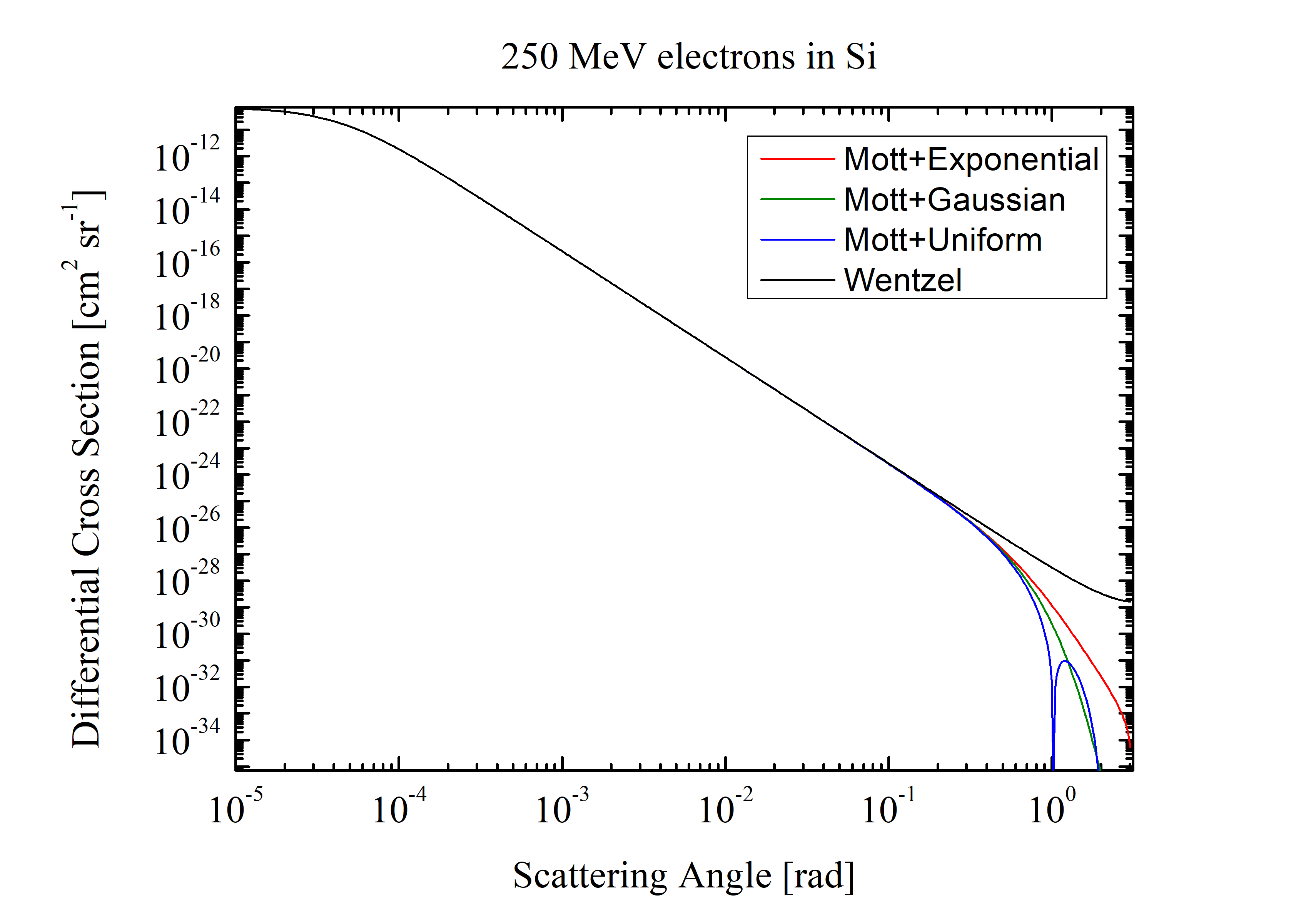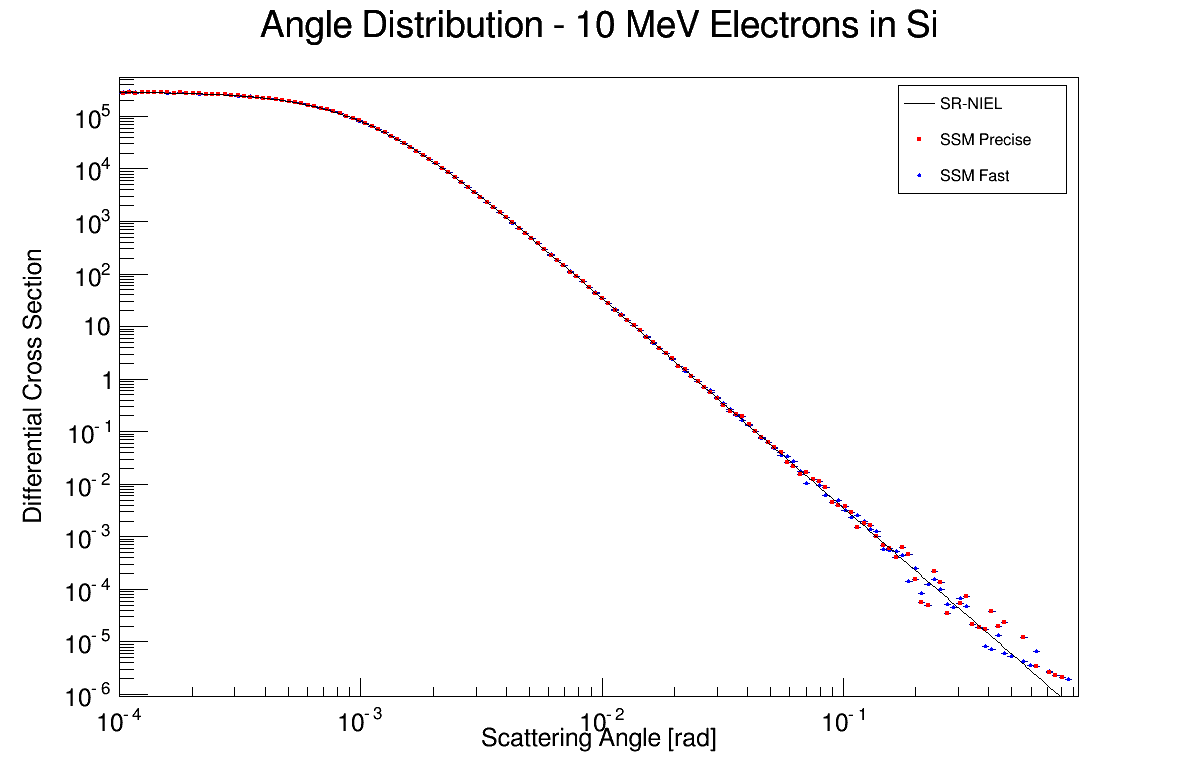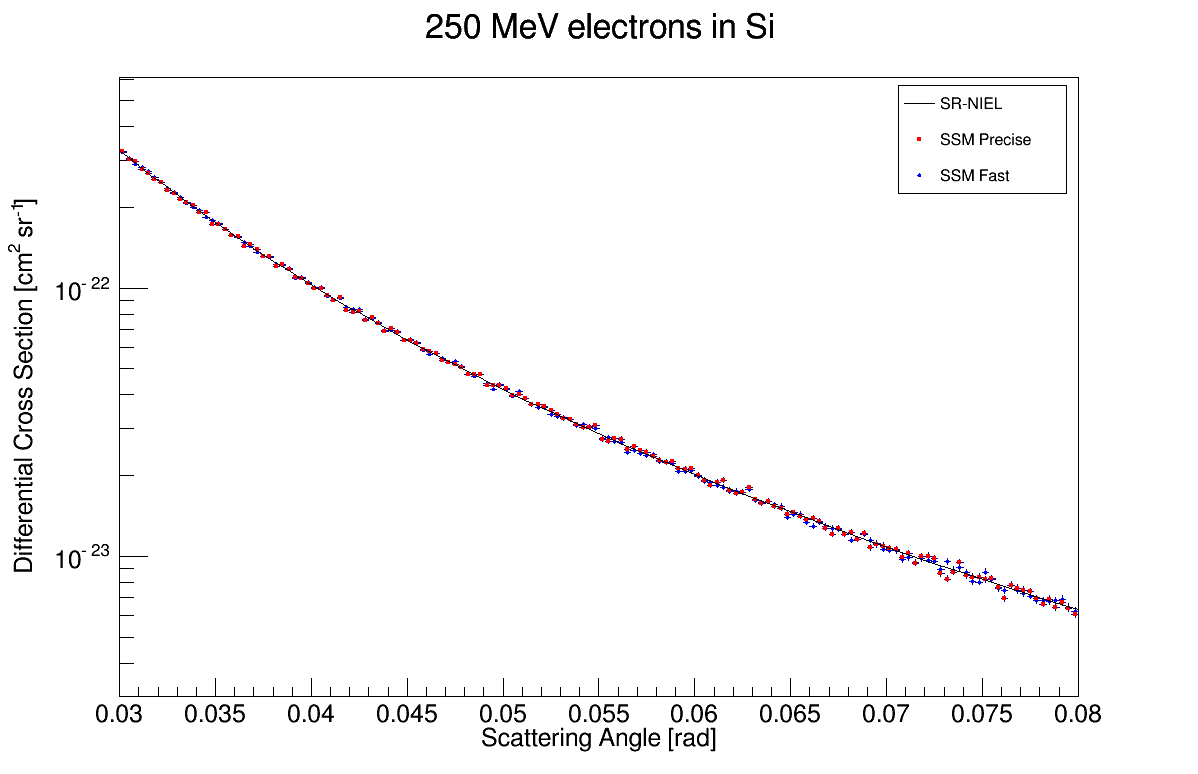Most of the computation time in the Geant4 electron scattering computation, impling SR-NIEL treatment, is due to the calculation of the Mott cross section value. Since Mott cross section is extracted from fit parameters, the integral function doesn't exist and the partial integral must be performed for every interaction.

Wentzel cross section is a good approximation of the Mott cross section for low scattering angle. Moreover Wentzel cross section is an anlytical function and its integral fuction exists. The calculation of the partial integral is much faster with Wentzel cross section with respect to the Mott cross section.

Wentzel cross section and Mott cross section are overlapped for very low scattering angle:Fig.1: Comparison of the Wentzel Cross section with respecto to the Mott cross section in case of different form factors.

A new, Fast, computational method is introduced in the new release of electron scattering class for Geant4.
Fast method, predefined in the initialization of the class, uses Wentzel Cross section for low scattering angles and when the difference from the Mott cross section is less than 3%. Mott cross section is used at high scattering angle.

Precise method uses only the Mott cross section for every scattering angle.

In the new release test58 is implemented a method to select precise (fast) computation in the macro file:

/testem/phys/setXSecModel precise
/testem/phys/setXSecModel fast

With low statistic simulations, when almost all the scattering occur at low angle, Fast method is 200 times faster than Precise method.

The new Fast method has been tested in comparison with Precise one, for low scattering angle and high scattering angle:Fig.2: Comparison of the Fast (red dots) and Precise (blue dots) results with respect to the SR-NIEL Mott Cross section as a function of the scattering angle. Precise method is using Mott cross section, Fast method is using Wentzel cross section.Fig.3: Comparison of the Fast (red dots) and Precise (blue dots) results with respect to the SR-NIEL Mott Cross section as a function of the scattering angle. Precise method is using Mott cross section, Fast method is using Wentzel cross section for angle lower than 0.038 rad and Mott cross section elsewhere. The transistion from one treatment to the other is smooth.

Hits: 923